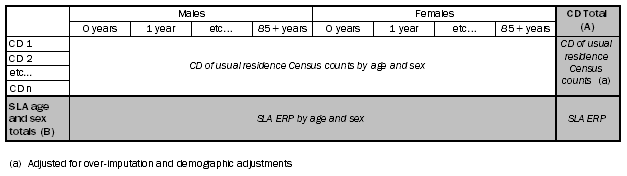3228.0.55.001 - Population Estimates: Concepts, Sources and Methods, 2009
Latest ISSUE Released at 11:30 AM (CANBERRA TIME) 12/06/2009
 Page tools: .mffeedback,#pjs { display :none; } Enable Javascript to Print PagesPrint AllEmail NotificationRSSSearch this Product APPENDIX 5 THE ITERATIVE PROPORTIONAL FITTING PROCEDURE INTRODUCTION A5.1 The iterative proportional fitting (IPF) procedure is used when reliable estimates for a desired cross-classification cannot be obtained directly, but estimates of the variables of interest, and possibly some related variables, are available at a higher level of aggregation. An additional requirement for the use of the IPF procedure is that information on the relationship between the variables is available at the desired level of cross-classification. A5.2 For example, estimated resident population (ERP) for Census collection districts (CDs) by age and sex cannot be calculated directly, as births, deaths and migration data are not available at the CD level by age and sex. However, we do have information on CD ERP totals (not by age and sex) and statistical local area (SLA) ERP by age and sex. This information may be used in an IPF to calculate CD ERP by age and sex. DATA REQUIREMENTS A5.3 In the IPF procedure, these two sources of information appear as two distinct classes of inputs known as the association structure and the allocation structure. The association structure, representing the relationship between available estimates, is typically a two-dimensional table of estimates, and the allocation structure consists of estimates of various 'marginals' of the table. (A 'marginal' of a table is the set of quantities obtained by adding across all categories of any one or more of the cross-classifying variables in the table). A5.4 In addition, the grand total may be a sum of one of the marginals, or it may be a separate figure. This is the figure to which all table cells (excluding marginals) will sum after the IPF procedure has been performed. PROCESS A5.5 The IPF procedure produces new estimates for each cell in the table by adjusting the initial estimates (the association structure) to agree with the marginal constraints provided by the allocation structure, in an iterative fashion. Method A5.6 For illustration, take the case where the association structure is a two-dimensional table, with two one-dimensional marginals. Step 1 A5.7 The column and row marginals are prorated and rounded so they sum to the grand total. Step 2 A5.8 The elements of each row of the table are prorated so their total equals the corresponding marginal estimate. Step 3 A5.9 The elements of each column are prorated so their total equals the corresponding estimate in the other marginal. Step 4 A5.10 As the estimates in the table no longer sum to the first marginal, steps 2 and 3 are repeated until the procedure converges to the unique solution which sums to the marginals while preserving the relationships as specified by the association structure. Step 5 A5.11 Population estimates and components of population change deal with whole numbers of persons, therefore after convergence, a rounding process that maintains the marginal totals is employed. Example A5.12 The technique is illustrated in table A5.1 where the CD ERP by age and sex is calculated using a two-dimensional IPF. A5.13 For Census year ERP, the initial estimates (matrix body) are CD of usual residence Census population counts by age and sex (with demographic adjustments applied), the column marginal (A) is the sum of these CD of usual residence population counts and the row marginal (B) is SLA ERP by age and sex from Population by Age and Sex, Regions of Australia (cat. no. 3235.0). A5.1 Calculation of CD ERP using iterative proportional fittingA5.14 In this case, only the column marginal is prorated to sum to the grand total as it was calculated by summing the row marginal. FURTHER INFORMATION A5.15 When an IPF procedure needs to be applied to a distribution with positive and negative values in either the association structure or the allocation structure, plus-minus pro ration (see Appendix 6 - The plus-minus proportional adjustment technique) is substituted for the standard method of pro ration. A5.16 For a more detailed description of the IPF procedure, see Siegel & Swanson (2004), pg 712. Document Selection These documents will be presented in a new window.